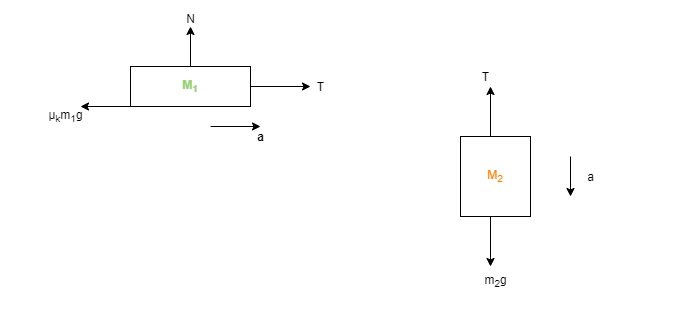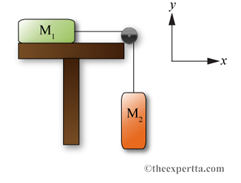# Problem: A block with mass m1 = 9.1 kg rests on the surface of a horizontal table which has a coefficient of kinetic friction of uk = 0.69. A second block with a mass m2 = 9.5 kg is connected to the first by an ideal pulley system such that the second block is hanging vertically. The second block is released and motion occurs. Part (a) Using the variable T to represent tension, write an expression for the sum of the forces in the y-direction, ΣFy, for block 2.Part (b) Using the variable T to represent tension, write an expression for the sum of the forces in the x-direction, ΣFx, for block 1.Part (c) Write an expression for the magnitude of the acceleration of block 2, a2, in terms of the acceleration of block 1, a1. (Assume the cable connecting the masses is ideal)Part (d) Write an expression using the variables provided for the magnitude of the tension force, TPart (e) What is the tension, T in Newtons?

###### FREE Expert Solution

The free-body diagram for the two masses:96% (12 ratings)###### Problem Details

A block with mass m= 9.1 kg rests on the surface of a horizontal table which has a coefficient of kinetic friction of uk = 0.69. A second block with a mass m2 = 9.5 kg is connected to the first by an ideal pulley system such that the second block is hanging vertically. The second block is released and motion occurs.Part (a) Using the variable T to represent tension, write an expression for the sum of the forces in the y-direction, ΣFy, for block 2.
Part (b) Using the variable T to represent tension, write an expression for the sum of the forces in the x-direction, ΣFx, for block 1.
Part (c) Write an expression for the magnitude of the acceleration of block 2, a2, in terms of the acceleration of block 1, a1. (Assume the cable connecting the masses is ideal)
Part (d) Write an expression using the variables provided for the magnitude of the tension force, T
Part (e) What is the tension, T in Newtons?